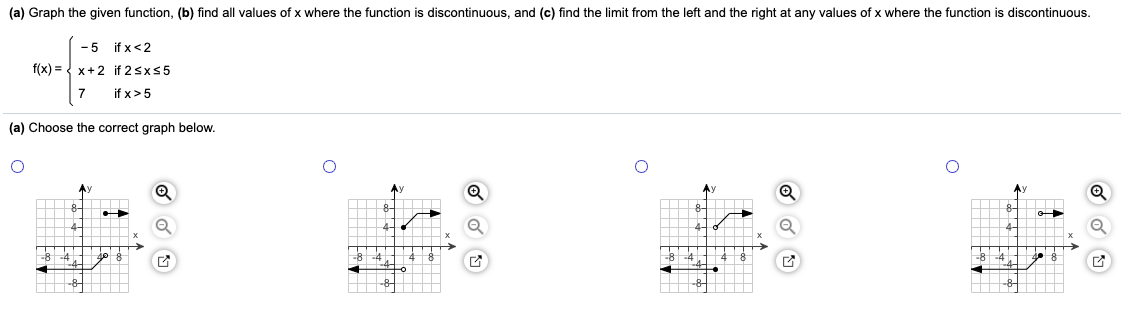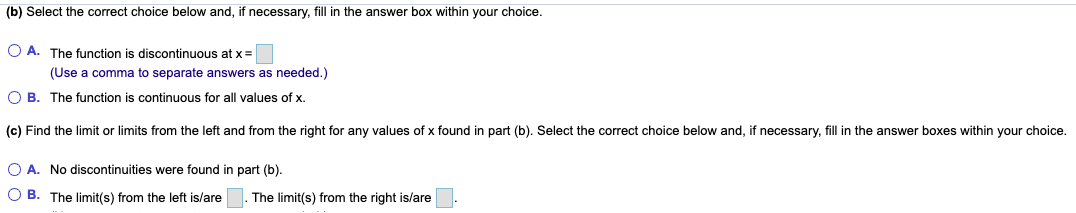Home / Answered Questions / Other / a-graph-the-given-function-b-find-all-values-of-x-where-the-function-is-discontinuous-and-c-find-the-aw934

# (Solved): (a) Graph The Given Function, (b) Find All Values Of X Where The Function Is Discontinuous, And (c) ...(a) Graph the given function, (b) find all values of x where the function is discontinuous, and (c) find the limit from the left and the right at any values of x where the function is discontinuous. ( -5 if x<2 f(x) = x+2 if 25x55 7 if x >5 (a) Choose the correct graph below. @ 8 (b) Select the correct choice below and, if necessary, fill in the answer box within your choice. O A. The function is discontinuous at x = (Use a comma to separate answers as needed.) B. The function is continuous for all values of x. O (c) Find the limit or limits from the left and from the right for any values of x found in part (b). Select the correct choice below and, if necessary, fill in the answer boxes within your choice. O A. No discontinuities were found in part (b). O B. The limit(s) from the left is/are . The limit(s) from the right is/are .

We have an Answer from Expert